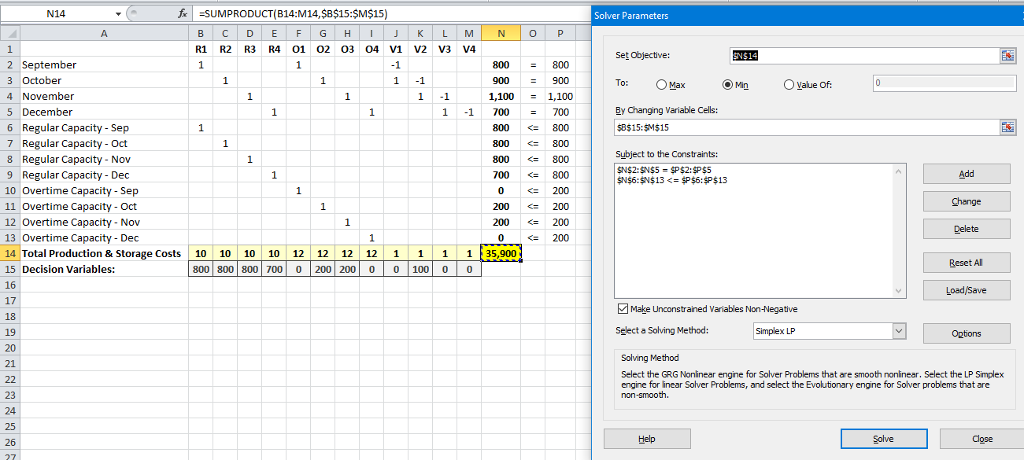# Question & Answer: The demand for a particular product in the next four months is expected to be as…..

The demand for a particular product in the next four months is expected to be as follows: September: 800 units, October: 900 units, November: 1100 units, and December 700 units. Operating during regular hours the production capacity is 800 units/month. But overtime capacity is an additional 200 units/month. The selling price is the same irrespective of production mode, and the unit production cost are \$10 and \$12 for regular time production and overtime production, respectively. In addition, there exists a storage cost of \$1 per unit per month for each unsold unit held over. Formulate this problem as a linear program to determine a production plan that minimizes total production and storage costs.

Decision Variables: R1, R2, R3, R4 = Regular production in each month

O1, O2, O3, O4 = Overtime production in each month

Don't use plagiarized sources. Get Your Custom Essay on
Question & Answer: The demand for a particular product in the next four months is expected to be as…..
GET AN ESSAY WRITTEN FOR YOU FROM AS LOW AS \$13/PAGE

V1, V2, V3, V4 = Inventory kept in storage in each month

Objective: Min 10(R1 + R2 + R3 + R4) + 12(O1 + O2 + O3 + O4) + 1(V1 + V2 + V3 + V4)

s.t.

R1 + O1 – V1 = 800

R2 + O2 + V1 – V2 = 900

R3 + O3 + V2 – V3 = 1100

R4 + O4 + V3 – V4 = 700

R1, R2, R3, R4, O1, O2, O3, O4, V1, V2, V3, V4 >= 0

Solution using Excel Solver is following